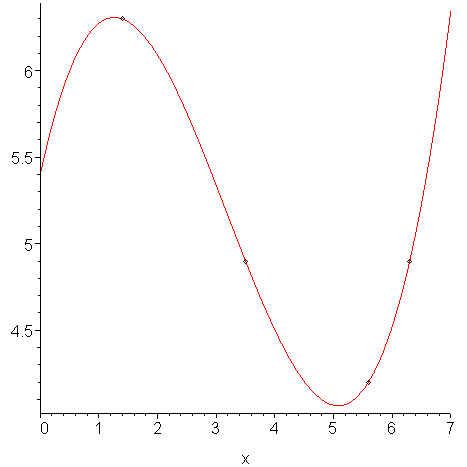## 5 Interpolation

Given a set of points (xi, yi) for i = 0, 1, 2, ..., n, we want to find a function (usually a polynomial) which passes through all of the points. For example, Figure 1 shows 4 points and a polynomial which passes through them.Figure 1. An interpolation of four points.

Often in engineering, you will only be able to sample points (usually periodically) and interpolation is one tool which can be used to estimate the values of points between those points which were sampled.

We will look at three techniques for finding interpolating polynomials:

1. The Vandermonde method (easy to calculate, easy to generalize)
2. Lagrange polynomials (easy to find by hand)
3. Newton polynomials (efficient to implement when using Horner's rule)

# The Third Tool

Interpolation also forms the third tool which we will use in developing other numerical techniques. After iteration, it forms the next most common tool. It is not as powerful as Taylor series, but is most useful when model information is either unavailable or too difficult to use efficiently.

# History

The first recorded attempts at quadratic interpolation begin with the Persian physicist Al-Biruni (973-1048) who was one of the earliest adopters of the scientific method; however, the first use of finite differences began with Sir Thomas Harriot (1560-1621) whose other legacy is the use of the symbols < and >. [Goldstine]